• ## ALU设计

千次阅读 2004-11-09 01:00:00
开始设计ALU加法的实现设计完毕，先行进位：P = A xor B ; G = A and B
开始设计ALU
加法的实现设计完毕，
先行进位：P = A xor B ; G = A and B
展开全文• ALU设计，包含源码及仿真等内容，比较简单，仅供参考。
• VHDL全加器设计以及ALU设计报告+（全代码）100%可以运行
• ## 实验案例-ALU设计

万次阅读 2019-02-15 17:40:56
实例四 ALU设计 2.4.1. 本章导读 ALU（算数逻辑单元）是CPU的基本组成部分。设计要求掌握算术逻辑运算加、减操作原理，验证运算器的组合功能。 设计原理 ALU的基本结构如图2-4-1所示。我们所设计的ALU要实现最...
实例四 ALU设计（基于Robei的实验案例）
2.4.1. 本章导读
ALU（算数逻辑单元）是CPU的基本组成部分。设计要求掌握算术逻辑运算加、减操作原理，验证运算器的组合功能。
设计原理
ALU的基本结构如图2-4-1所示。我们所设计的ALU要实现最基本的加减运算，与或非和异或等功能。（1）加法运算包含2种类型，一种是不带进位的加法器，另外一种是带进位的加法器。不带进位的加法器的公式：
{D,R}=A+B                                       (1)
带进位的可以进行加法器级联，实现更高位数的串行加法运算。带进位的加法器的公式：
{D,R}=A+B+F                                    (2)
（2）减法运算也包含2种类型。不带借位的减法运算：
{D,R}=A-B                                          (3)
带借位的减法运算：
{D,R}=A-B-F                                       (4)
设计要求
设计一个8位ALU，并能实现数据与，或，非，异或，不带进位加法，带进位加法，不带借位减法和带借位减法运算。运算符采用3比特表示。A，B，R 均为8比特数据。用测试文件测试你的ALU功能，并用级联方式将4个8比特的ALU实现32 比特的ALU。
2.4.2. 设计流程
1. ALU模型设计

新建一个模型命名为alu，类型为module，同时具备4输入2输出。每个引脚的属性和名称参照图2-4-2进行对应的修改。图2-4-2 引脚属性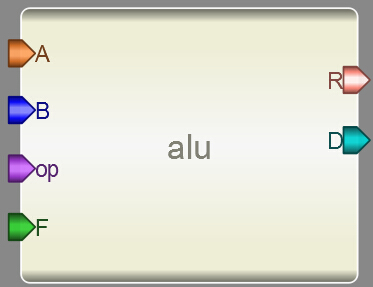图2-4-3 ALU界面图
添加代码。点击模型下方的Code（如图2-4-4所示）添加代码。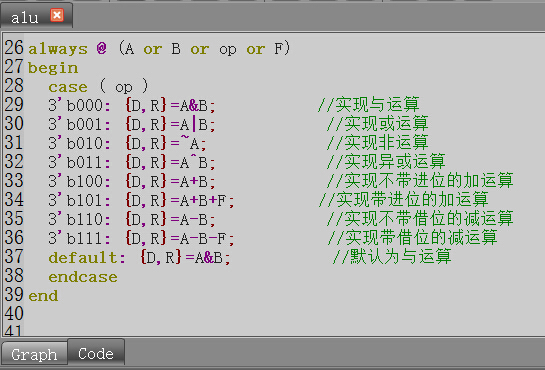图2-4-4 点击Code输入算法
在代码设计区内输入以下Verilog代码：
always @ (A or B or op or F)
begin
case ( op )
3’b000: {D,R}=A&B;           //实现与运算
3’b001: {D,R}=A|B;            //实现或运算
3’b010: {D,R}=~A;             //实现非运算
3’b011: {D,R}=A^B;            //实现异或运算
3’b100: {D,R}=A+B;            //实现不带进位的加运算
3’b101: {D,R}=A+B+F;         //实现带进位的加运算
3’b110: {D,R}=A-B;            //实现不带借位的减运算
3’b111: {D,R}=A-B-F;          //实现带借位的减运算
default: {D,R}=A&B;           //默认为与运算
endcase
end

（3）保存模型到一个文件夹中，运行并检查有无错误输出。
2. 测试文件设计
（1）新建一个4输入2输出的测试文件，记得将Module Type设置为“testbench”各个引脚配置如图2-4-5所示。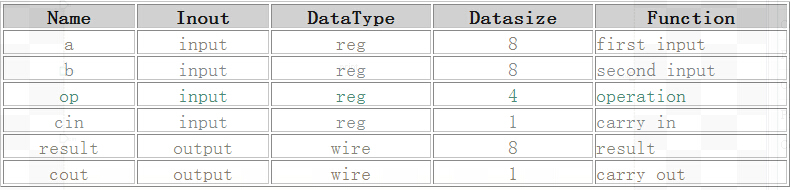图2-4-5 新建测试文件
（2）另存为测试文件。将测试文件保存到alu模型所在的文件夹下。
（3）加入模型。在Toolbox工具箱的Current栏里，会出现一个alu模型，单击该模型并在alutest上添加，并连接引脚，如图2-4-6所示。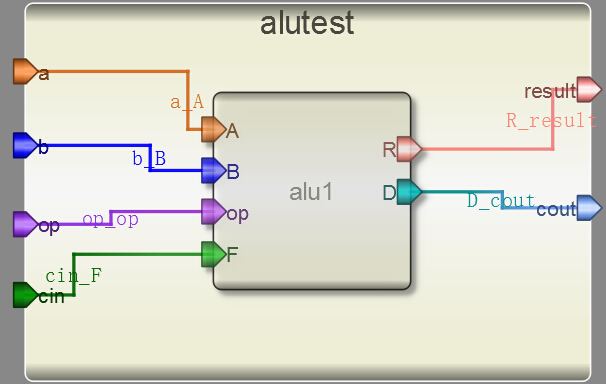图2-4-6 添加模型
（4）输入激励。点击测试模块下方的“Code”，输入激励算法，如图2-4-7所示。激励代码在结束的时候要用$finish 结束。 initial begin a=0; b=0; op=0; cin=0; #1 a=3; b=1; op=0; #1 a=2; b=1; op=1; #1 a=255; b=0; op=2; #1 a=5; b=6; op=3; #1 a=128; b=128; op=4; #1 a=4; b=5; cin=1; op=5; #1 a=4; b=5; op=6; #1 a=4; b=5; op=7; #1 a=4; b=5; op=0; #1$finish;
end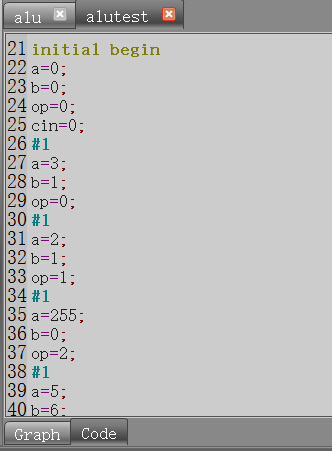图2-4-7 激励代码
（5）执行仿真并查看波形。查看输出信息。检查没有错误之后查看波形。点击右侧Workspace中的信号，进行添加并查看分析仿真结果，如图2-4-8所示。对照真值表，查看设计波形输入输出是否一致。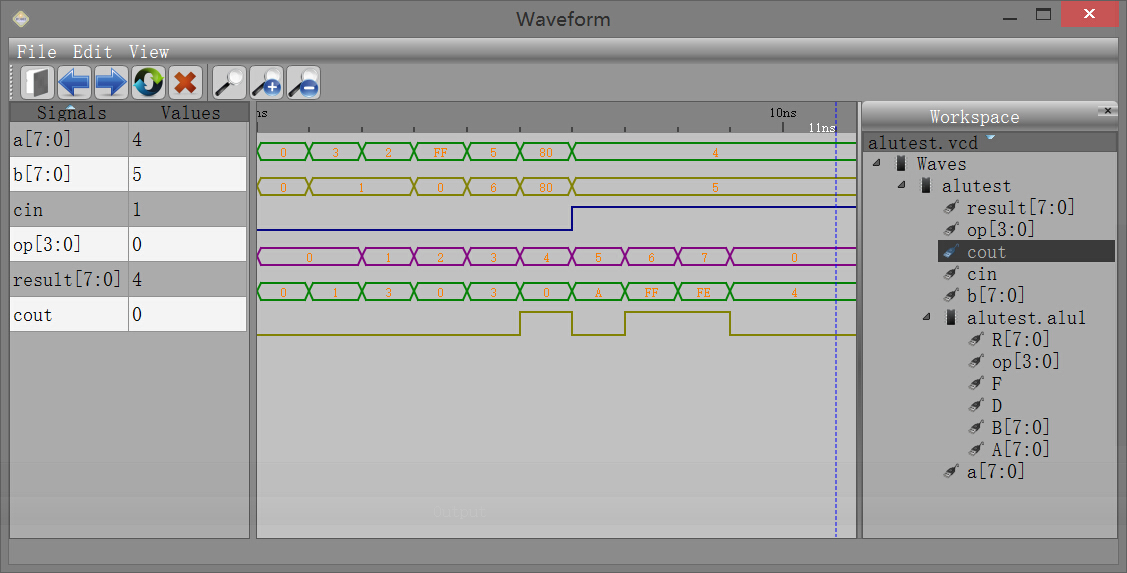图2-4-8 查看波形
3. 16位ALU设计
（1）下面我们来设计一个16位的ALU。这个设计中使用之前设计好的8位ALU模块，把输入的16位信号拆分为两个8位信号，经过8位ALU处理后再组合成最终的16位输出信号。
（2）split模块设计：该模块的功能是把输入的16位数据分解为两个8位数据。模块引脚设计如图2-4-9所示。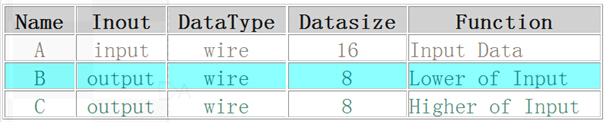图2-4-9 split模块引脚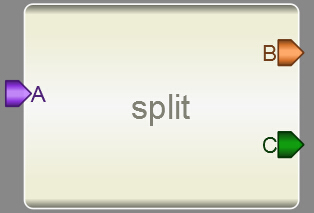图2-4-10 split模块设计
模块设计好后，点击code标签，输入split模块的代码：
assign B=A[7:0];
assign C=A[15:8];
（3）merge模块设计：该模块的功能是把两个8位数据组合为一个16位数据。模块引脚设计如图2-4-11所示。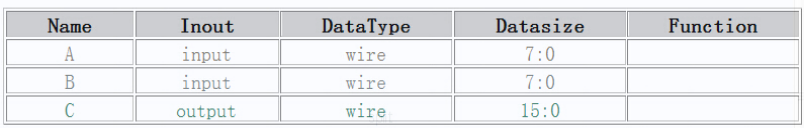图2-4-11 merge模块引脚图2-4-12 merge模块设计
模块设计好后，点击code标签，输入merge模块的代码：
assign C={B, A};
（4）接下来建立一个模块，命名为alu16，具有4输入和2输出，引脚设定如下图2-4-13所示：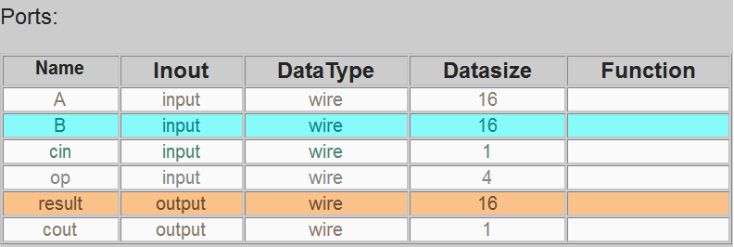图2-4-13 alu16模块引脚设计
保存之后把之前设计好的ALU，split和merge模块添加进alu16模块，并进行连线。完成后的模块如图2-4-14所示：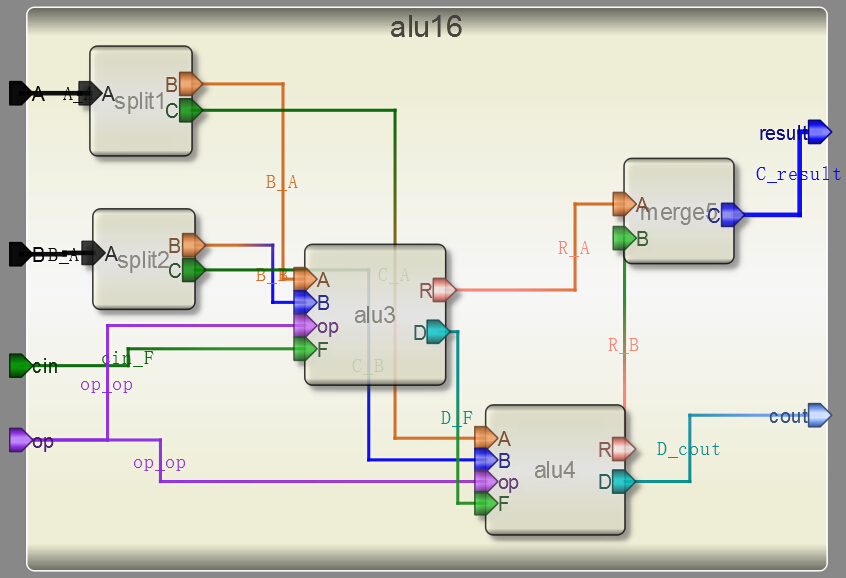图2-4-14  alu16模块设计
（5）测试模块设计：新建一个模块，模块类型选择为testbench，引脚设计如图2-4-15所示。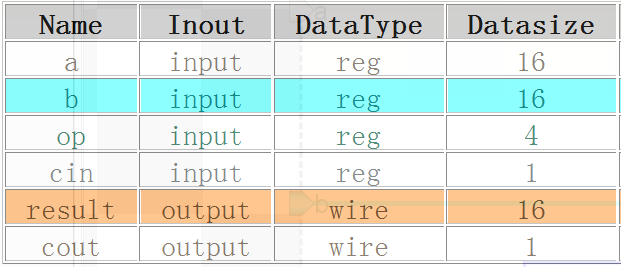图2-4-15  alu16的测试模块引脚设计
保存后把之前设计的alu16模块添加进测试模块，并进行连线。连线后的测试模块如图2-4-16所示。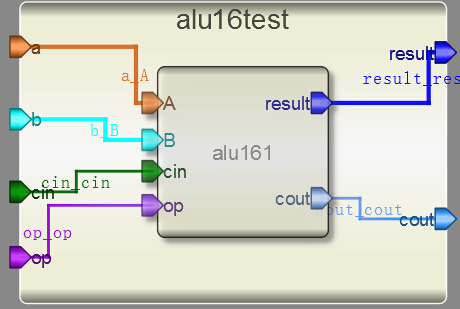图2-4-16 alu16的测试模块设计
点击code标签，输入测试模块的激励代码：
initial begin
a=0;
b=0;
op=0;
cin=0;
#1
a=24;
b=35;
op=0;
#1
a=56;
b=18;
op=1;
#1
a=96;
b=80;
op=2;
#1
a=51;
b=26;
op=3;
#1
a=128;
b=128;
op=4;
#1
a=64;
b=15;
cin=1;
op=5;
#1
a=74;
b=35;
op=6;
#1
a=24;
b=75;
op=7;
#1
a=24;
b=55;
op=0;
#1
$finish; end （6）运行后，点击Wave查看波形，如图2-4-17所示，检查设计的正确性。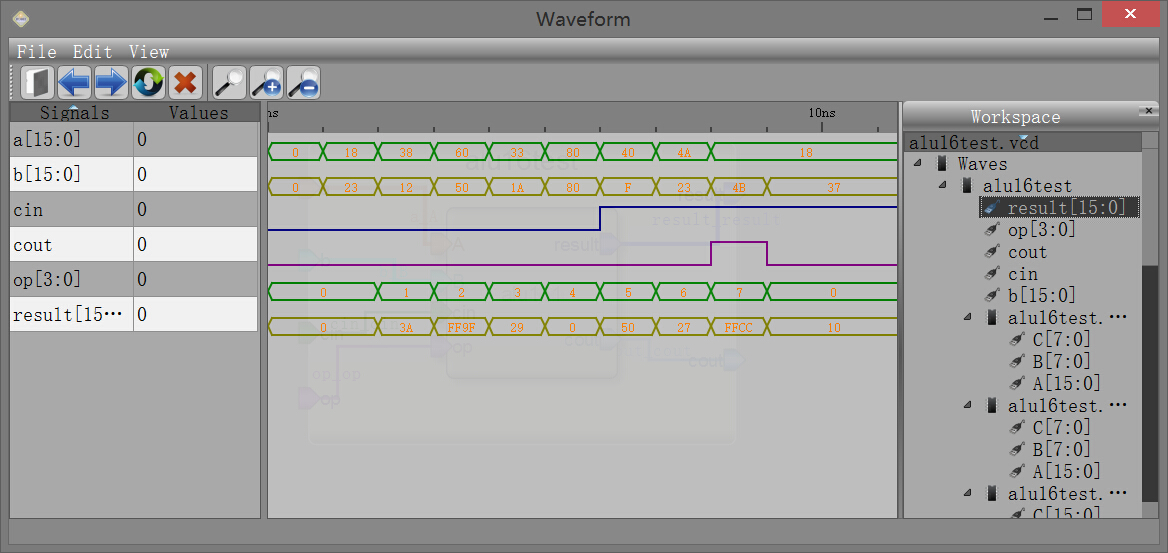图2-4-17 alu16的测试模块仿真波形 4. 32位ALU设计 （1）我们利用8位的ALU级联来设计一个32位的ALU，这个设计需要先行注册Robei软件，否则不能进行仿真。 （2）创建一个新的模型，添加10个输入引脚，5个输出引脚，各个引脚的配置如图2-4-18所示。保存到alu模型所在的文件夹。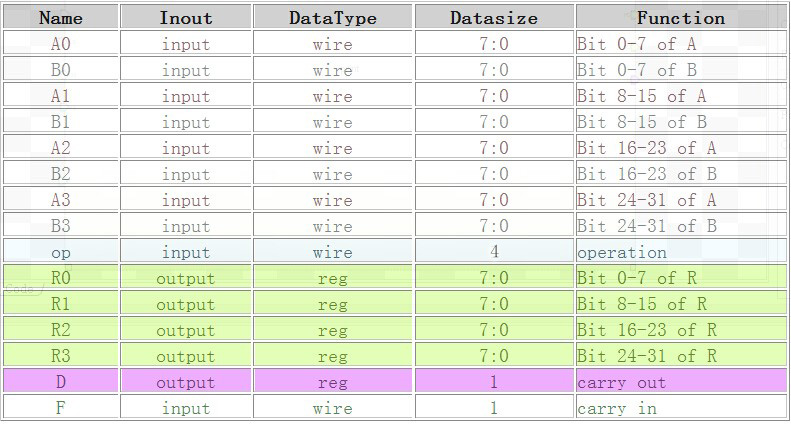图2-4-18 32位ALU引脚 （3）添加4个ALU连接引脚。如图2-4-19所示。4个8位的ALU进行级联，第一个输出的D连到下一级的F，最终的ALU的D连接到顶层的D引脚。第一个ALU的F连接到顶层模块的F。op都连接到顶层的op引脚上，A，B和R按照高低位进行连接。这样输入A3A2A1A0，B3B2B1B0和R3R2R1R0分别是32位ALU的输入和输出端。如图2-4-19所示。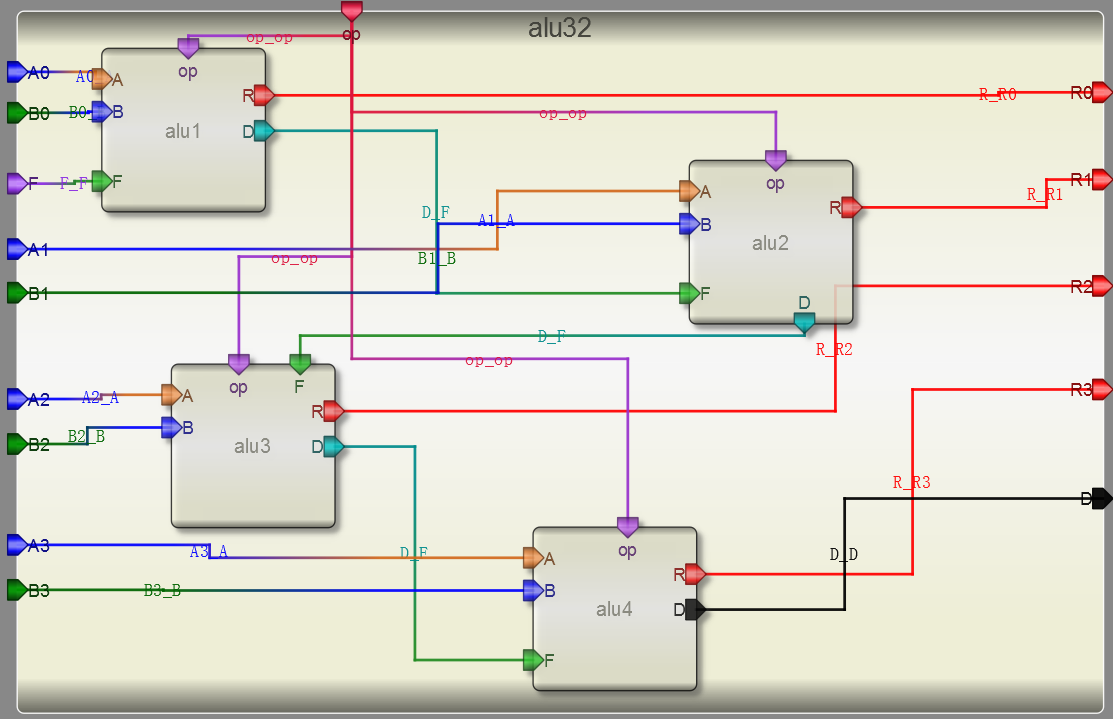图2-4-19 32位ALU设计图 （4）创建一个测试文件，10个输入引脚5个输出，按照图2-4-20进行引脚配置并保存到与alu32bit模型同一个文件下。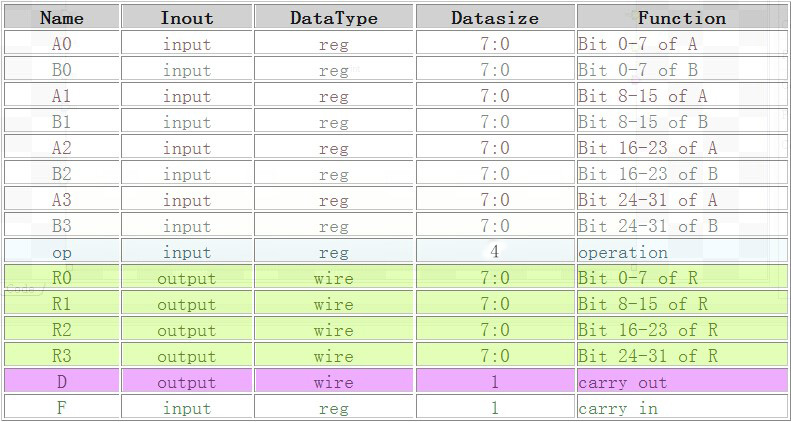图2-4-20 32位ALU测试文件引脚配置 （5）从Toolbox里面的Current栏找alu32bit模型，并添加到测试模块上。对应引脚相连。如图2-4-21所示。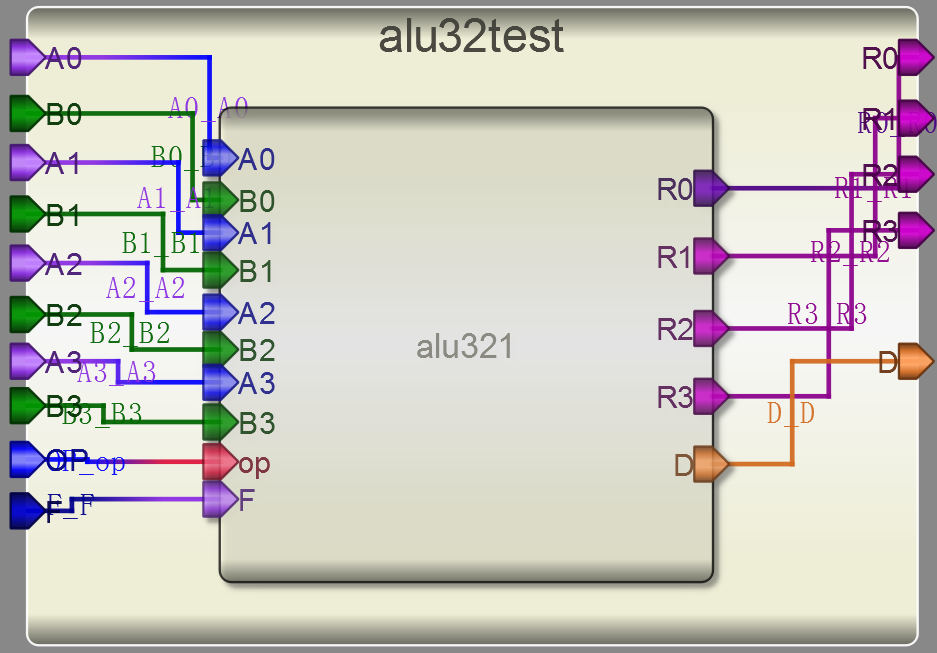图2-4-21 32位ALU测试引脚连接 （6）自己设计测试激励代码，并仿真查看结果。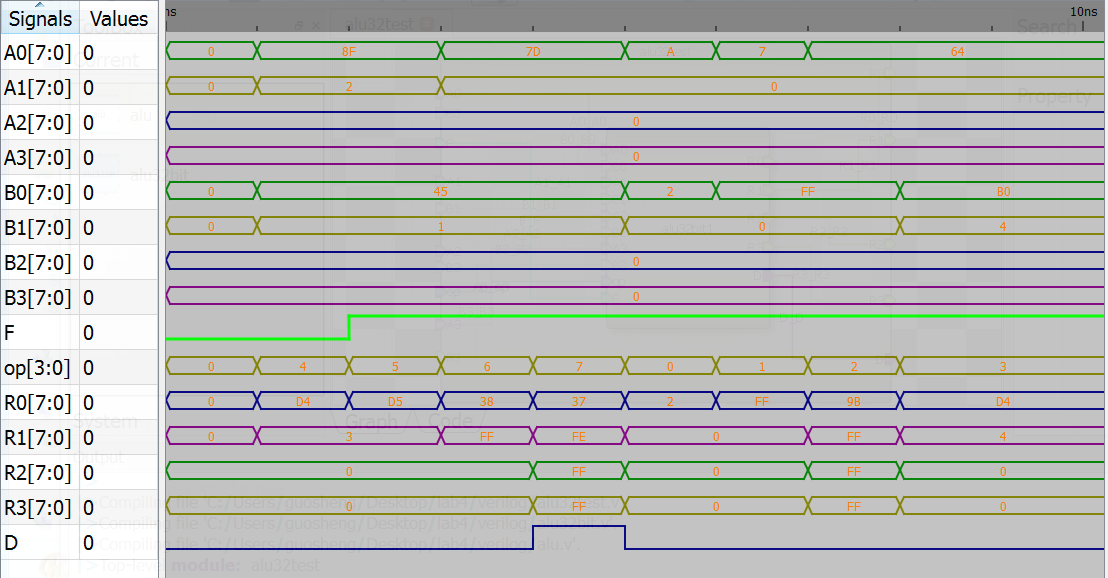图2-4-22 32位ALU仿真波形 2.4.3. 问题与思考 1、不要使用8位ALU级联的方式，尝试直接用Verilog在Robei中实现一个32位或者64位ALU。 2、挑战题：在8位ALU设计上添加乘法功能，输出结果变成16位输出。利用这个ALU实现一个16位的乘法器。提示：16位乘法器分成低8位和高8位。如A[15:0]拆分成A[15:8]和A[7:0]，同样拆分B。之后用4个乘法器分别实现： A[7:0]×B[7:0] A[7:0]×B[15:8] A[15:8]×B[7:0] A[15:8]×B[15:8] 然后进行适当移位，再用加法器实现相加。  展开全文FPGA案例 • 8位可控加减法器设计、32位算术逻辑运算单元ALU设计、四位先行进位74182、四位快速加法器 、8位快速加法器、16位快速加法器、5位阵列乘法、6位补码阵列乘法器等电路，已画好。alu自动测试是100分。 • ## 32位ALU设计(verilog实现) 千次阅读 多人点赞 2020-11-06 18:40:42 32位ALU设计 ​ 算术逻辑单元(arithmetic and logic unit) 是能实现多组算术运算和逻辑运算的组合逻辑电路，简称ALU。算术逻辑单元是中央处理器(CPU)的执行单元，是所有中央处理器的核心组成部分，由"And Gate"... 32位ALU设计 ​ 算术逻辑单元(arithmetic and logic unit) 是能实现多组算术运算和逻辑运算的组合逻辑电路，简称ALU。算术逻辑单元是中央处理器(CPU)的执行单元，是所有中央处理器的核心组成部分，由"And Gate"（与门） 和"Or Gate"（或门）构成的算术逻辑单元，主要功能是进行二位元的算术运算，如加减乘(不包括整数除法)。基本上，在所有现代CPU体系结构中，二进制都以补码的形式来表示。 设计思路 设计实现 仿真测试 文章目录32位ALU设计一、设计思路1. 算术运算2. 逻辑运算3.比较运算4.移位运算二、设计实现三、仿真测试四、模块设计1. CLA_adder32(1). 简单实现(2). 超前进位加法器(Carry-lookahead adder) 一、设计思路 1. 算术运算 addu、subu addu: 最高有效位向高位有进位，产生进位carryout subu: 当被减数小于减数时，最高有效位向高位有借位，产生借位carryout add、sub [x]补+[y]补=[x+y]补 [x-y]补=[x]补-[y]补=[x]补+[-y]补 [-y补]=~[y]补+1 add溢出：两个正数相加产生负数0111(7)+0110(6)=1101 ​ 两个负数相加产生正数1000(-8)+1001(-7)=0001 ​ 一个正数与一个负数相加不产生溢出 sub溢出：正数 - 正数（不产生溢出） ​ 正数 - 负数，结果为负数（上溢） ​ 负数 - 负数（不产生溢出） ​ 负数 - 正数，结果为正数（下溢） 2. 逻辑运算 ~：按位取反 &(and)：按位与操作 |(or)：按位或操作 ^(xor)：按位异或操作 ~(|)(nor)：按位或非操作 指令对标志位影响：指令执行后，CF和OF置0，ZF根据结果是否为0设置 3.比较运算 有符号数比较 slt 指令对标志位影响：in0小于in1置CF为1 in0为正数，in1为负数，out为0 in0为负数，in1为正数，out为1 in0和in1为负数，alu进行补码运算，1111（-1）、1110（-2），直接进行数值比较的结果与其代表的有符号数比较结果相同 in0和in1为正数，直接进行比较 通过分析，后两种情况可以合并 无符号数比较 sltu 可直接进行比较 指令对标志位影响：in0小于in1置OF为1 4.移位运算 逻辑左移shl (shift logical left)：sll，sllv 将数据向左移动，最低位用0补充 逻辑右移shr (shift logical right)：srl，srlv 将数据向右移动，最高位用0补充 算术右移sar (shift arithmetic right)：sra，srav 将各位依次右移指定位数，然后在左侧用原符号位补齐 指令对标志的影响：将最后移出的移位写入CF 总结来说，这六条移位操作指令可以分为两种情况：sllv、srav、srlv这3条指令的助记符最后有“v”，表示移位位数是通过寄存器的值确定的，sll、sra、srl这3条指令的助记符最后没有“v”，表示移位位数就是指令中6-10bit的sa的值。通过循环的方式，将移位后还在[31:0]部分的位置移到新的rd中，其他位置补上应补的0或1。 二、设计实现 module ALU32( op,in0,in1, carryout,overflow,zero,out ); input [31:0] in0,in1; input [10:0] op; output reg[31:0] out; output reg carryout,overflow,zero; always@(*) begin case(op) //add 11'b00000100000: begin out=in0+in1; overflow=((in0==in1)&&(~out==in0))?1:0; zero=(out==0)?1:0; carryout=0; end //addu 11'b00000100001: begin {carryout,out}=in0+in1; zero=(out==0)?1:0; overflow=0; end //sub 11'b00000100010: begin out=in0-in1; overflow=((in0==0&&in1==1&&out==1)|| (in0==1&&in1==0&&out==0))?1:0; zero=(in0==in1)?1:0; carryout=0; end //subu 11'b00000100011: begin {carryout,out}=in0-in1; zero=(out==0)?1:0; overflow=0; end //and 11'b00000100100: begin out=in0&in1; zero=(out==0)?1:0; carryout=0; overflow=0; end //or 11'b00000100101: begin out=in0|in1; zero=(out==0)?1:0; carryout=0; overflow=0; end //xor 11'b00000100110: begin out=in0^in1; zero=(out==0)?1:0; carryout=0; overflow=0; end //nor 11'b00000100111: begin out=~(in0|in1); zero=(out==0)?1:0; carryout=0; overflow=0; end //slt 11'b00000101010: begin if(in0==1&&in1==0) out=1; else if(in0==0&&in1==1) out=0; else out=(in0<in1)?1:0; overflow=out; zero=(out==0)?1:0; carryout=0; end //sltu 11'b00000101011: begin out=(in0<in1)?1:0; carryout=out; zero=(out==0)?1:0; overflow=0; end //shl 11'b00000000100: begin {carryout,out}=in0<<in1; overflow=0; zero=(out==0)?1:0; end //shr 11'b00000000110: begin out=in0>>in1; carryout=in0[in1-1]; overflow=0; zero=(out==0)?1:0; end //sar 11'b00000000111: begin out=($signed(in0))>>>in1;
carryout=in0[in1-1];
overflow=0;
zero=(out==0)?1:0;
end

endcase
end
endmodule

module ALU32_test(

);
reg [10:0] op;
reg [31:0] in0,in1;
wire [31:0] out;
wire carryout,overflow,zero;
ALU32 alu(op,in0,in1,carryout,overflow,zero,out);
initial
begin
//add
op=11'b00000100000;
in0=32'hf2340000;
in1=32'h80000000;
#20  in0=32'h7fffffff;
in1=32'h70000001;
#20  in0=32'h7fffffff;
in1=32'hf0000001;
#20  in0=32'hffffffff;
in1=32'h00000001;
//addu
#20  op=11'b00000100001;
in0=32'hf2340000;
in1=32'h80000000;
#20  in0=32'h7fffffff;
in1=32'h70000001;
#20  in0=32'hffffffff;
in1=32'h00000001;
//sub
#20  op=11'b00000100010;
in0=32'h72340000;
in1=32'h60000000;
#20  in0=32'h7fffffff;
in1=32'hf0000001;
#20  in0=32'hf00fffff;
in1=32'h7ffffff1;
#20  in0=32'hffffffff;
in1=32'hffffffff;
#20  in0=32'hf0000000;
in1=32'h0fffffff;
//subu
#20  op=11'b00000100011;
in0=32'h72340000;
in1=32'h60000000;
#20  in0=32'h7fffffff;
in1=32'hf0000001;
#20  in0=32'hffffffff;
in1=32'hffffffff;
#20  in0=32'hf0000000;
in1=32'h0fffffff;
//and
#20  op=11'b00000100100;
in0=32'h72340000;
in1=32'h60000000;
#20  in0=32'h7fffffff;
in1=32'h00000000;
//or
#20  op=11'b00000100101;
in0=32'h00000000;
in1=32'h00000000;
#20  in0=32'h7fffffff;
in1=32'hf0000001;
//xor
#20  op=11'b00000100110;
in0=32'ha0000000;
in1=32'h50000000;
#20  in0=32'h7fffffff;
in1=32'hf0000001;
//nor
#20  op=11'b00000100111;
in0=32'h123451ff;
in1=32'h60000000;
#20  in0=32'h7fffffff;
in1=32'hf0000001;
//slt
#20  op=11'b00000101010;
in0=32'h72340000;
in1=32'hf0000000;
#20  in0=32'h7000000f;
in1=32'h7f000001;
#20  in0=32'hf0001231;
in1=32'h7ac34545;
//sltu
#20  op=11'b00000101011;
in0=32'h72340000;
in1=32'hf0000000;
#20  in0=32'h7000000f;
in1=32'h7f000001;
#20  in0=32'hf0001231;
in1=32'h7ac34545;
//shl
#20  op=11'b00000000100;
in0=32'hffffffff;
in1=32'd5;
//shr
#20  op=11'b00000000110;
in0=32'hffffffff;
in1=32'd5;
//sar
#20  op=11'b00000000111;
in0=32'hffffffff;
in1=32'd3;
#20  in0=32'h0fffffff;
in1=32'd5;
end

endmodule

三、仿真测试

add、addu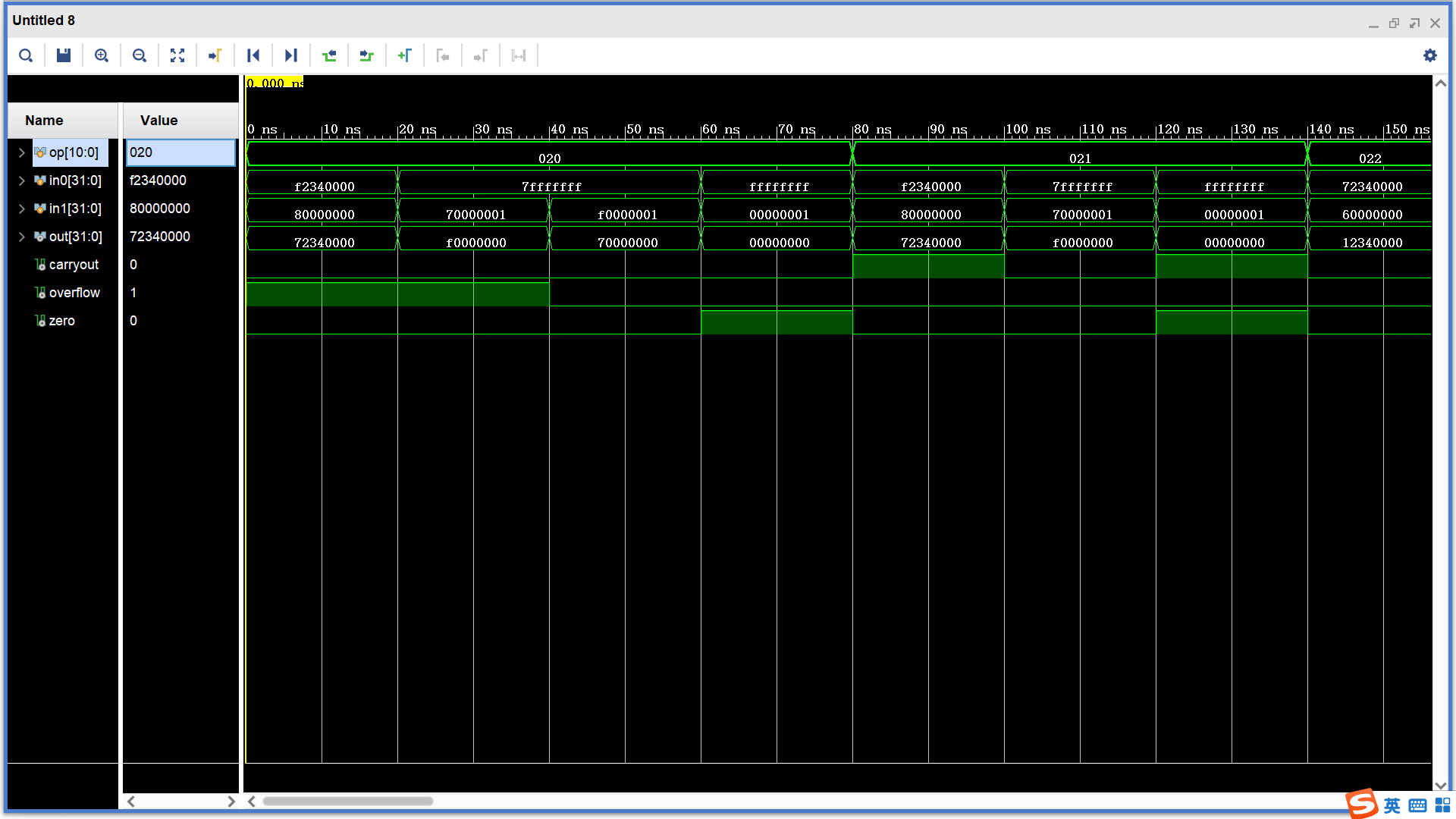sub、subu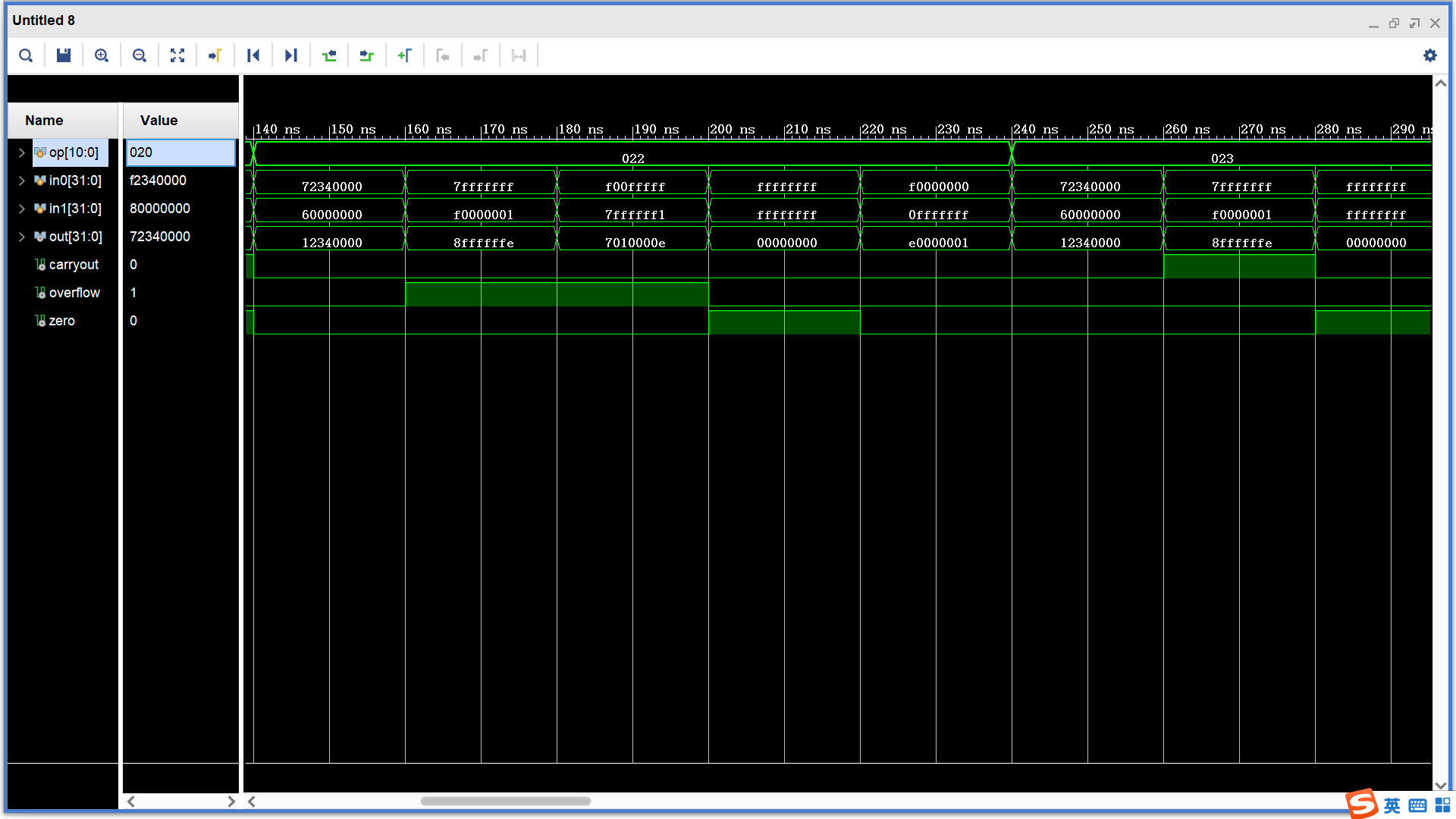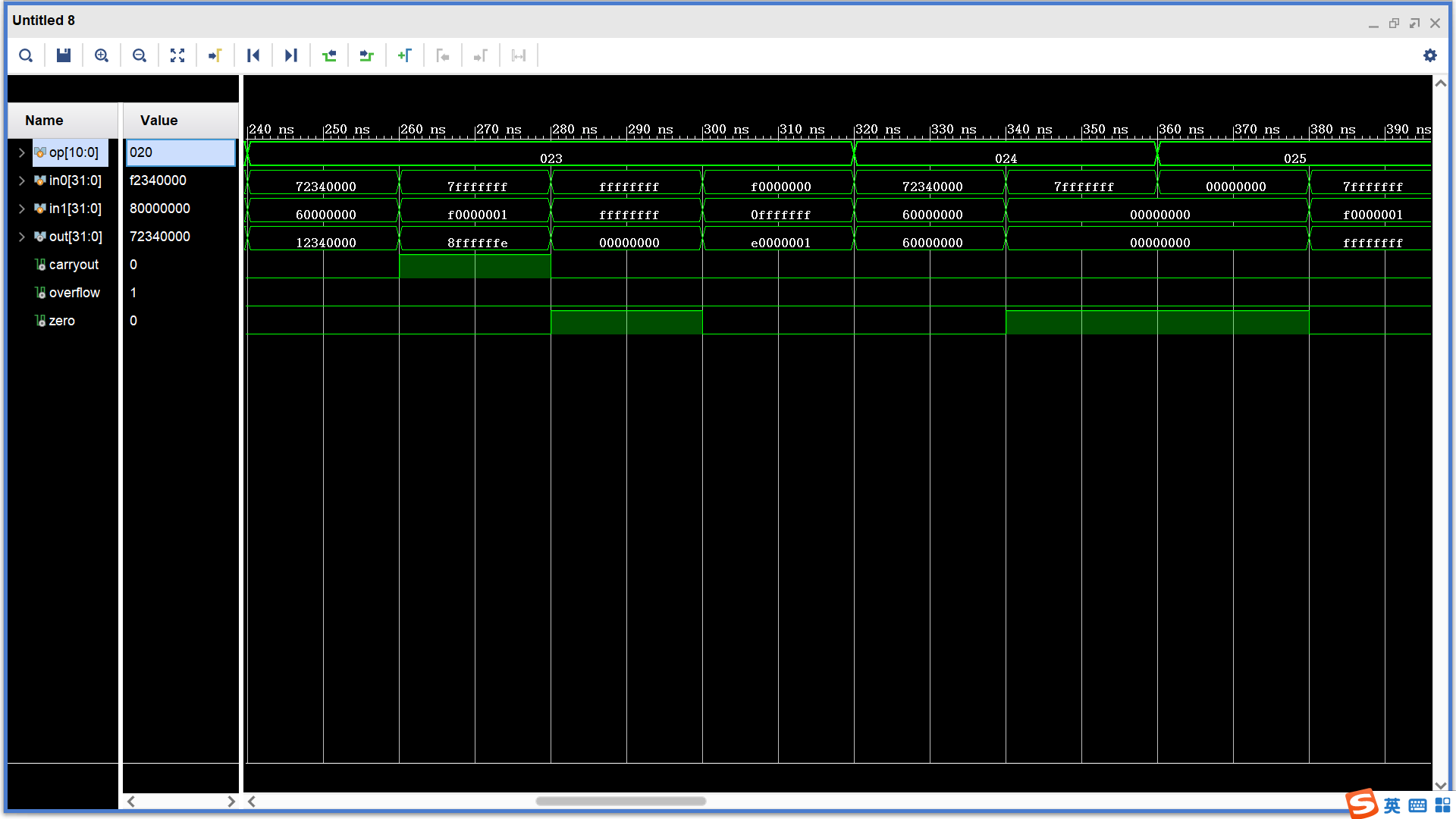and、or、xor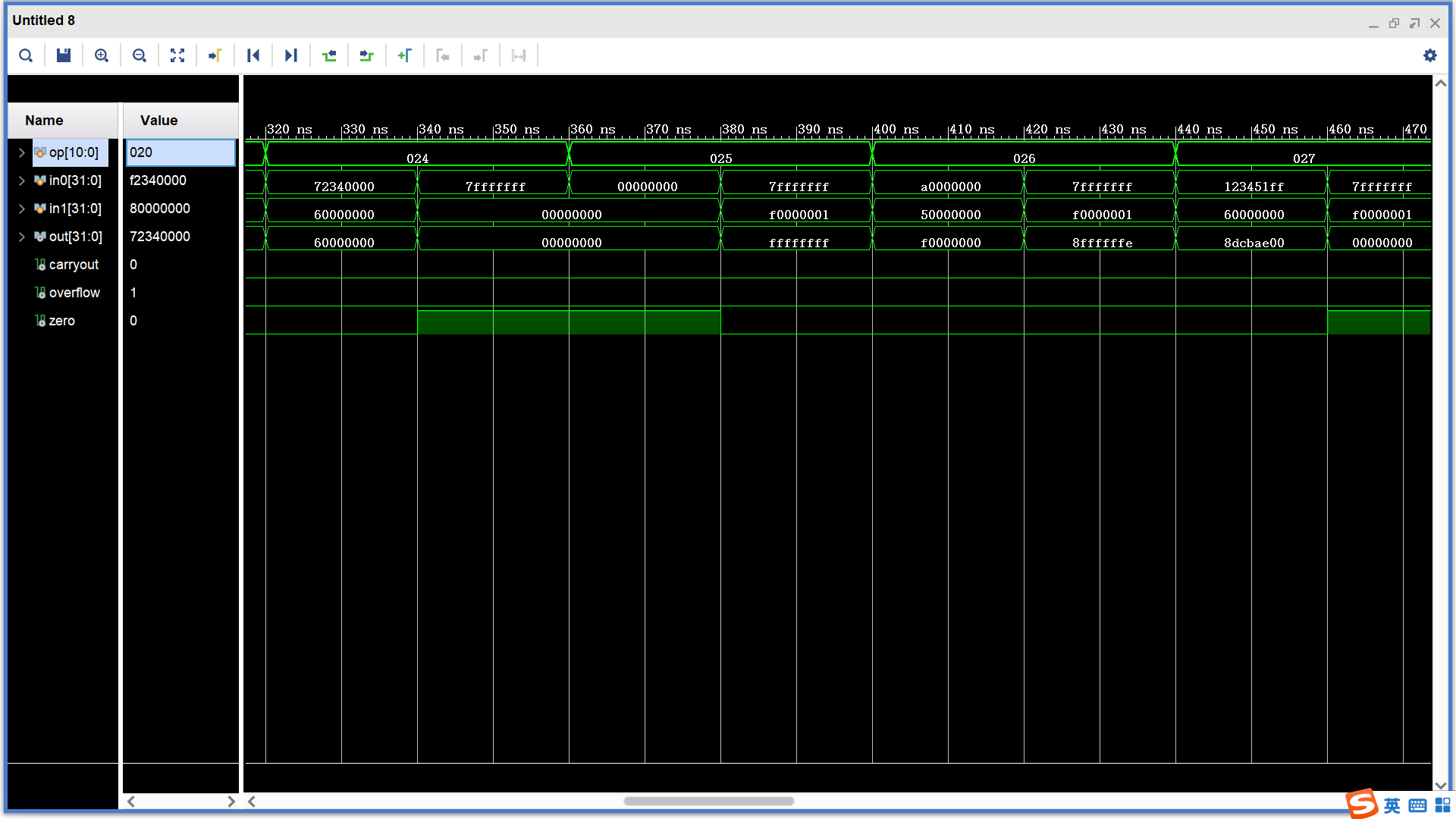nor、slt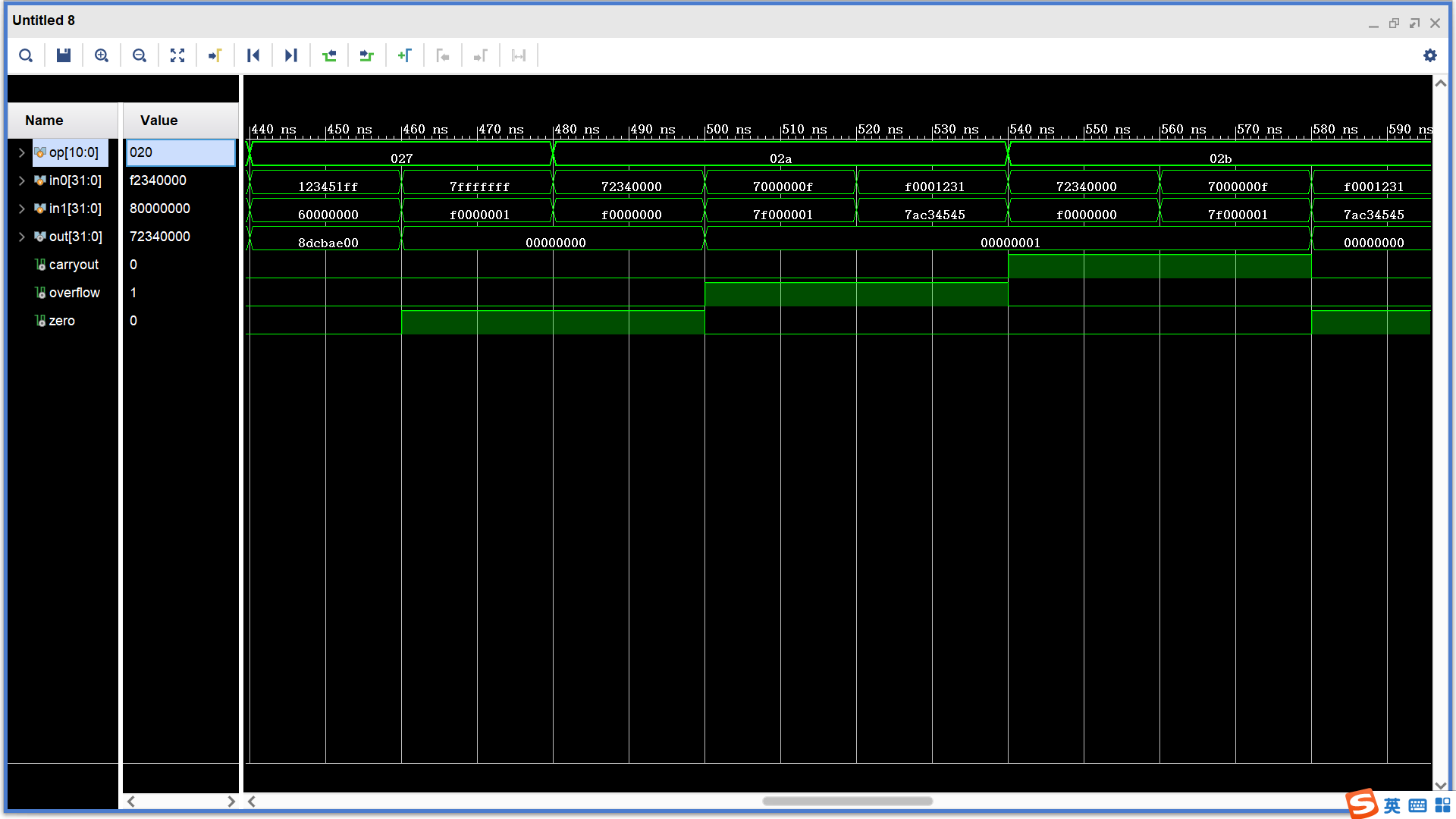sltu、shl、shr、sar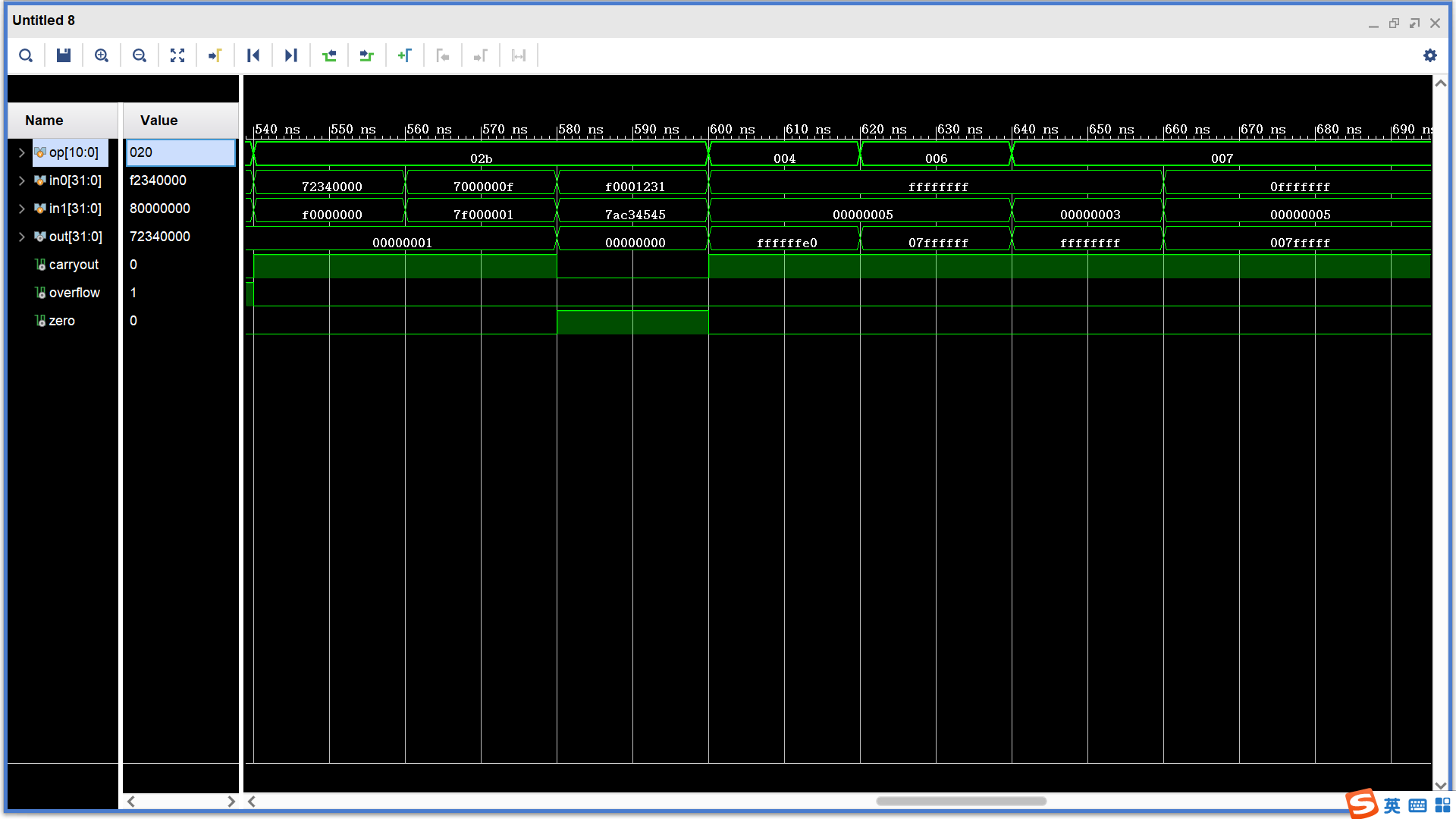四、模块设计
1. CLA_adder32
(1). 简单实现
  module full_adder(
cin,x,y,cout,s
);
input [31:0] x,y;
input cin;
output cout;
output [31:0] s;
assign {cout,s}=cin+x+y;
endmodule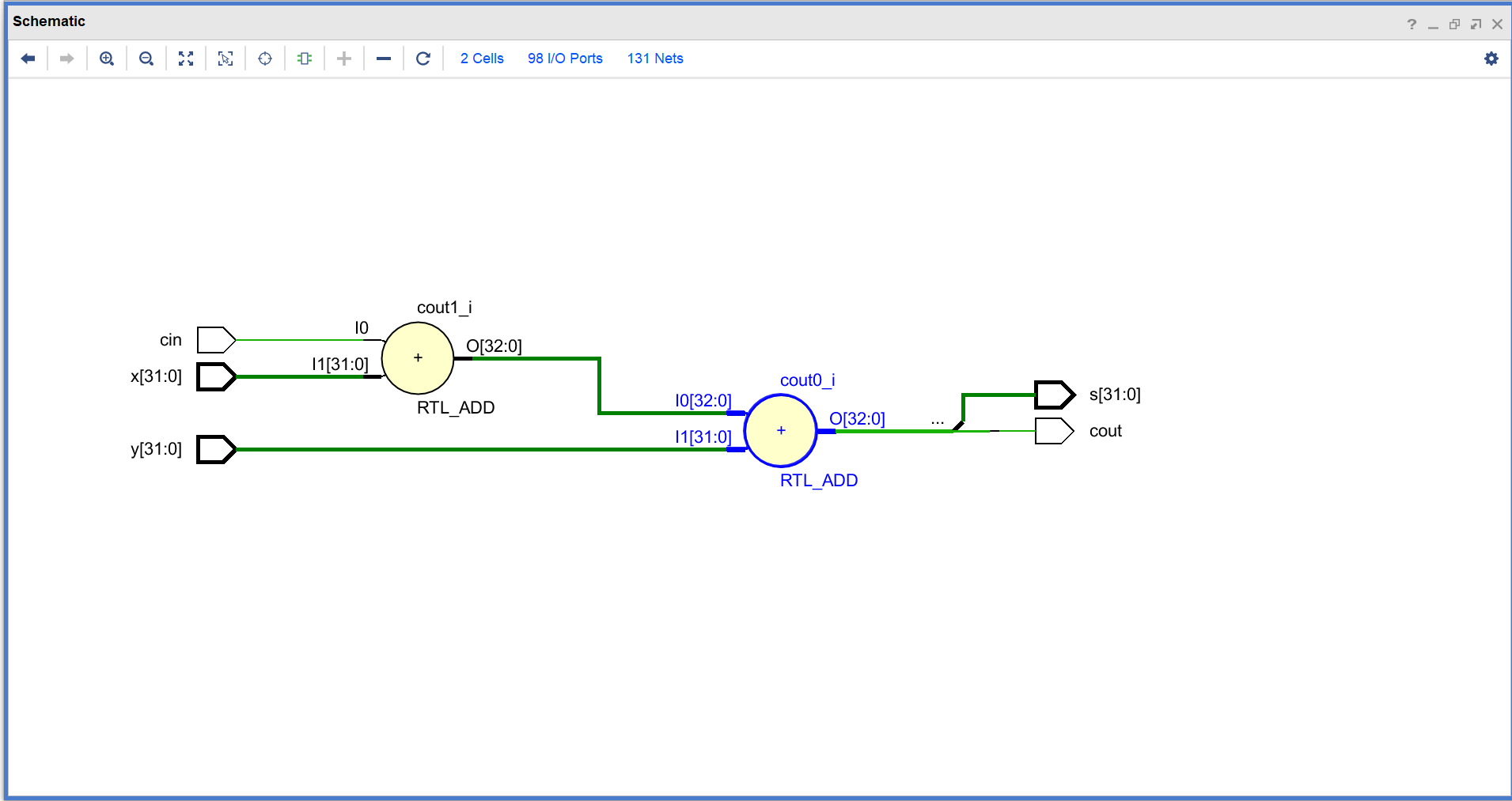这里在综合后使用了两个32位全加器，实际门延迟比32位全加器高。
(2). 超前进位加法器(Carry-lookahead adder)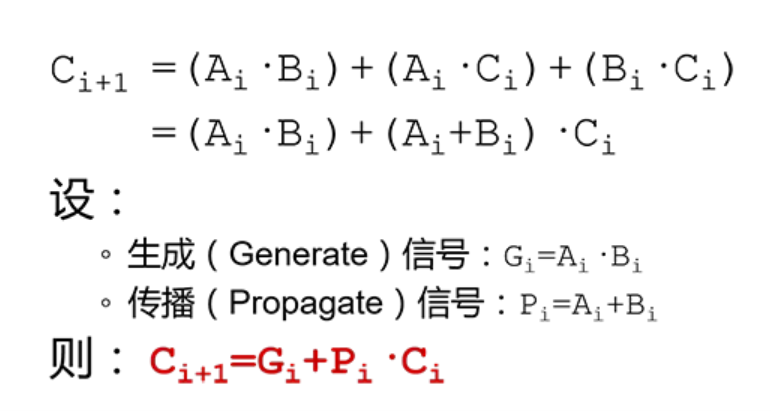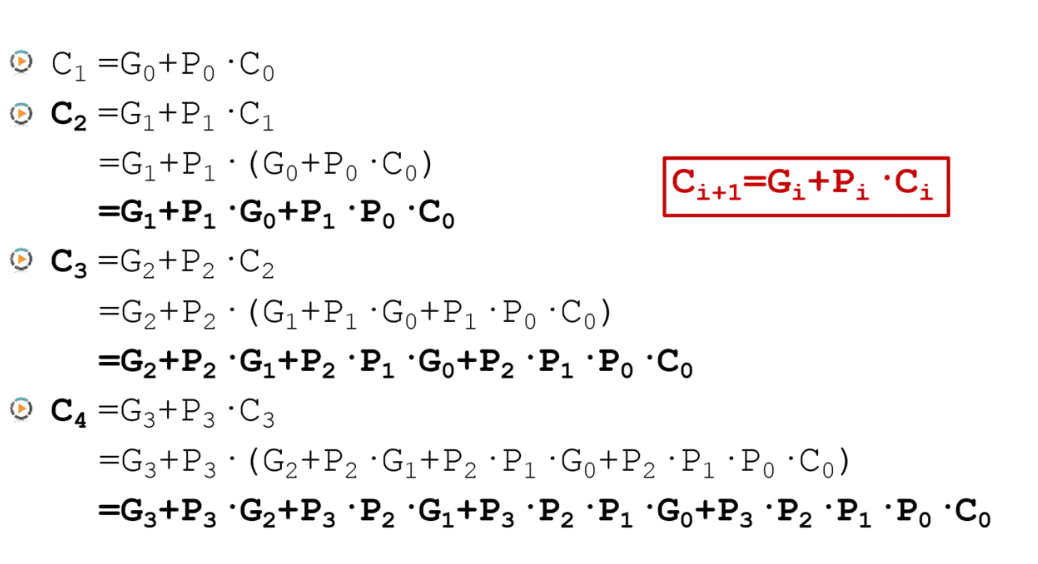加法器的优化——超前进位加法器
由上式可以看出直接实现32位超前进位加法器会非常复杂，所以首先实现8位超前进位加法器，并通过4个8位超前进位加法器组成32位超前进位加法器。
module CLA_adder8(
cin,a,b,
cout,s
);
input [7:0] a,b;
input cin;
output [7:0] s;
output cout;
wire [7:0] G,P;
wire [7:0] C;

assign G=a&b;
assign P=a|b;
assign C=cin;
assign s=a^b^C;

assign G=a&b;
assign P=a|b;
assign C=G|(P&cin);
assign s=a^b^C;

assign G=a&b;
assign P=a|b;
assign C=G | (P&G) | (P&P&cin);
assign s=a^b^C;

assign G=a&b;
assign P=a|b;
assign C=G | (P&G) | (P&P&G) | (P&P&P&cin);
assign s=a^b^C;

assign G=a&b;
assign P=a|b;
assign C=G | (P&G) | (P&P&G) | (P&P&P&G) |    						(P&P&P&P&cin);
assign s=a^b^C;

assign G=a&b;
assign P=a|b;
assign C=G | (P&G) | (P&P&G) | (P&P&P&G) | 						(P&P&P&P&G) |
(P&P&P&P&P&cin);
assign s=a^b^C;

assign G=a&b;
assign P=a|b;
assign C=G | (P&G) | (P&P&G) | (P&P&P&G) | 						(P&P&P&P&G) |
(P&P&P&P&P&G) | (P&P&P&P&P&P&cin);
assign s=a^b^C;

assign G=a&b;
assign P=a|b;
assign C=G | (P&G) | (P&P&G) | (P&P&P&G) | 						(P&P&P&P&G) |(P&P&P&P&P&G) | 							(P&P&P&P&P&P&G) |
(P&P&P&P&P&P&P&cin);
assign s=a^b^C;

assign cout=G | (P&G) | (P&P&G) | (P&P&P&G) | 						(P&P&P&P&G) |(P&P&P&P&P&G) | 							(P&P&P&P&P&P&G) |
(P&P&P&P&P&P&P&G) |
(P&P&P&P&P&P&P&P&cin);
endmodule

仿真测试：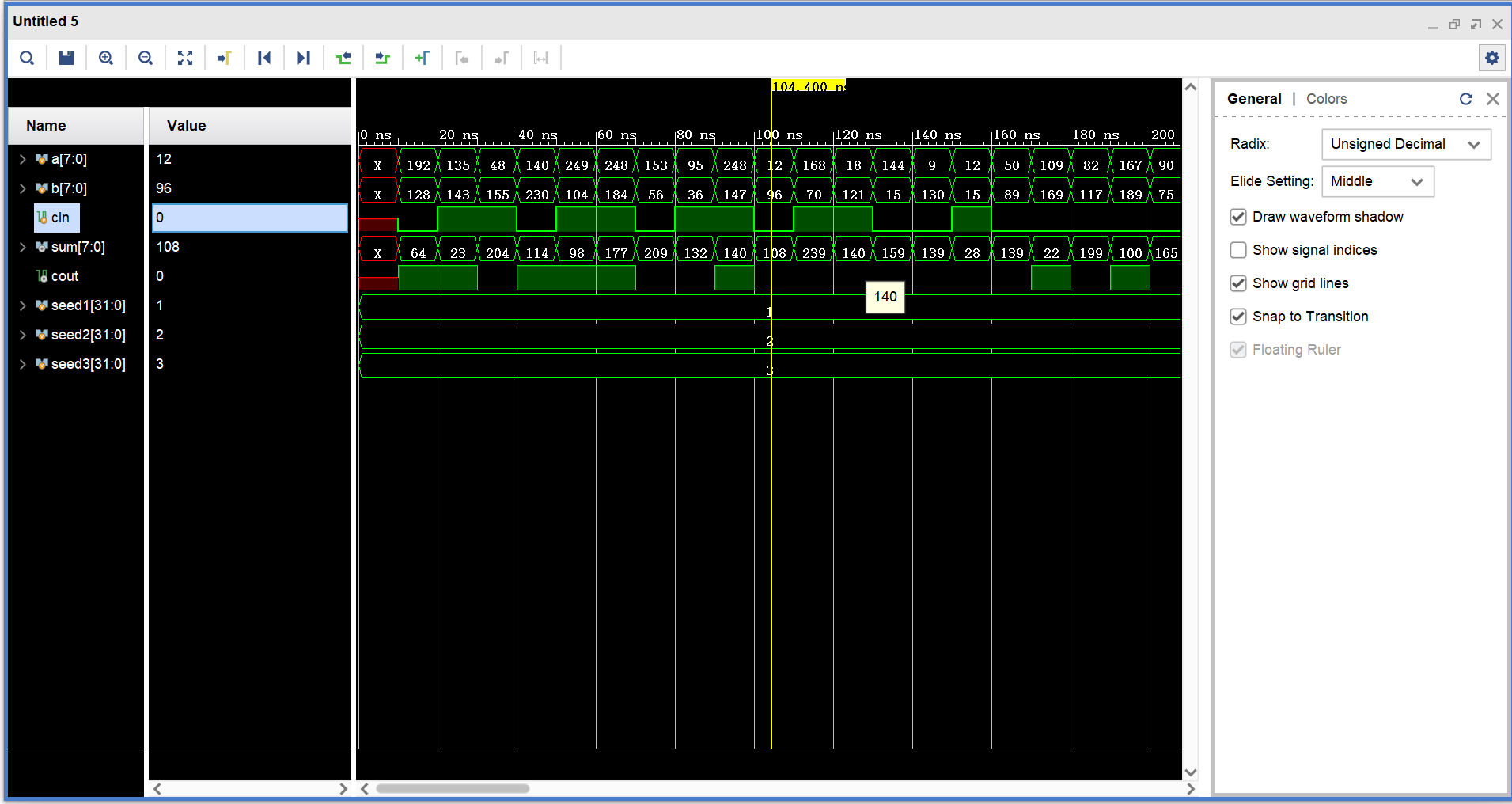门延迟分析：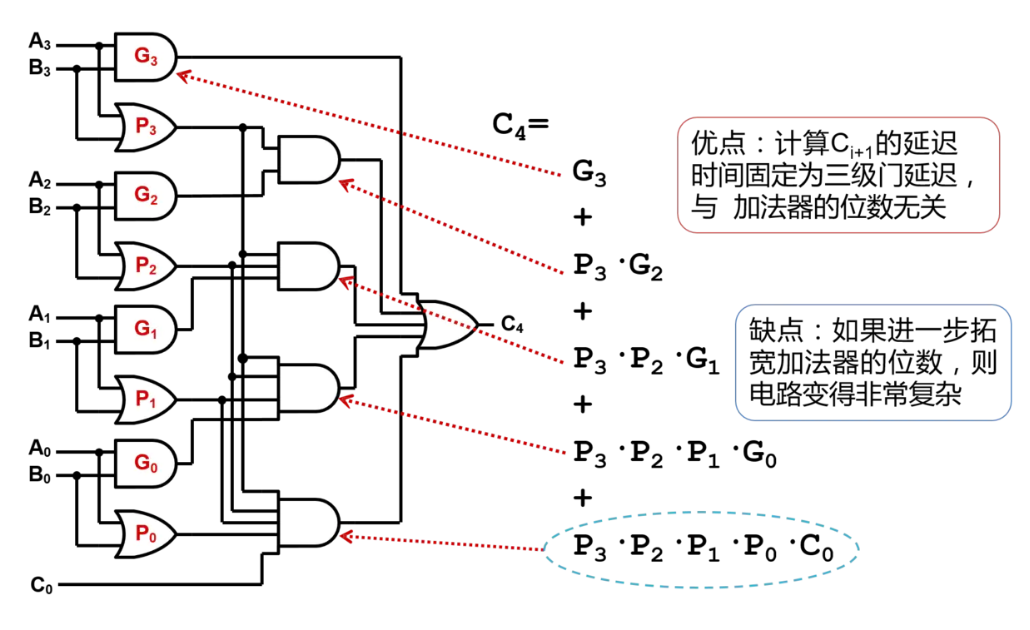4级8位超前进位加法器构成的32位超前进位加法器门延迟：3*4+1=13
module CLA_adder32(
cin,x,y,
cout,s
);
input [31:0] x,y;
input cin;
output [31:0] s;
output cout;
wire cout1,cout2,cout3;
CLA_adder8 step1(cin,x[7:0],y[7:0],cout1,s[7:0]);
CLA_adder8 step2(cout1,x[15:8],y[15:8],cout2,s[15:8]);
CLA_adder8 step3(cout2,x[23:16],y[23:16],cout3,s[23:16]);
CLA_adder8 step4(cout3,x[31:24],y[31:24],cout,s[31:24]);
endmodule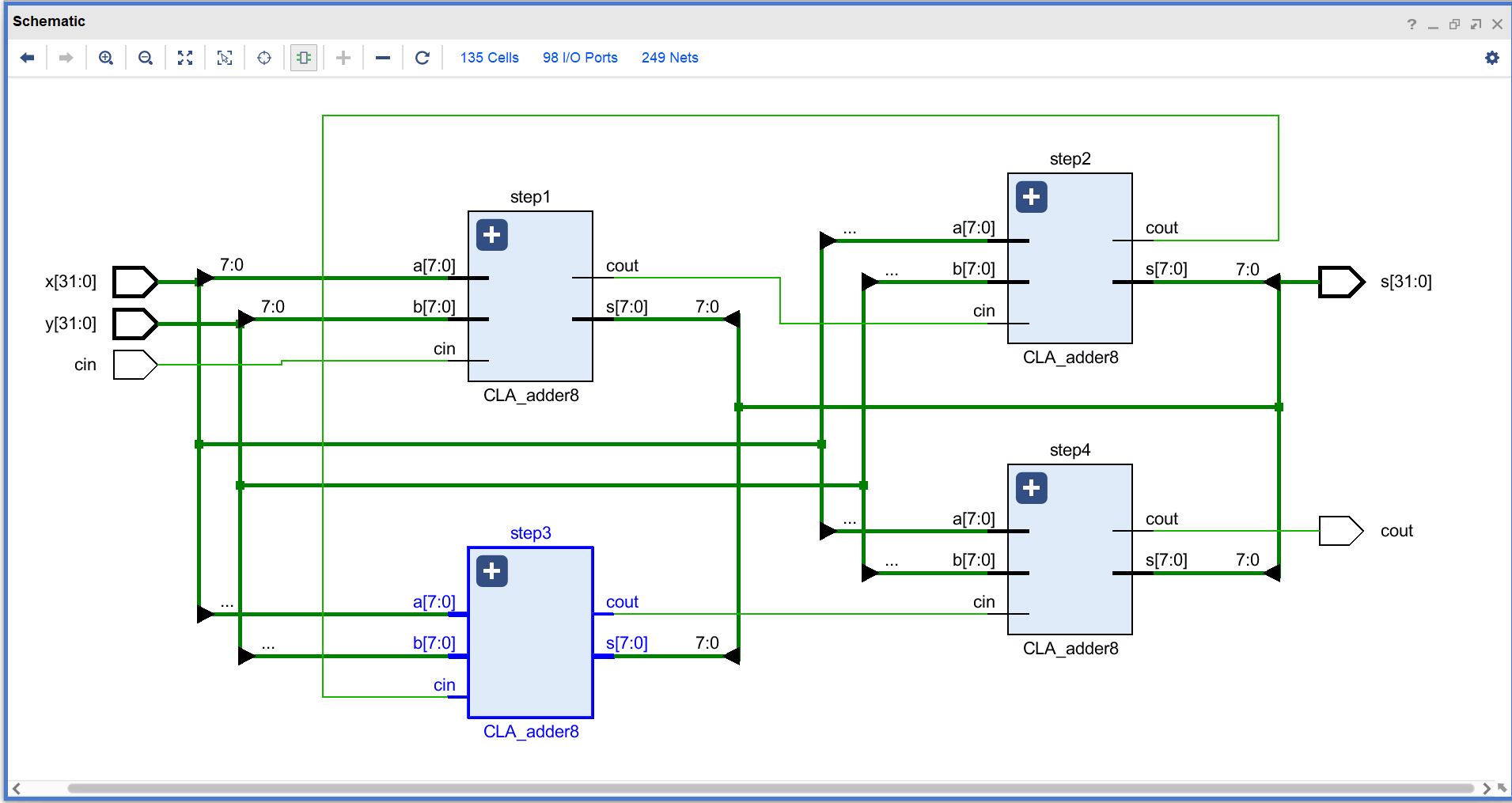仿真测试：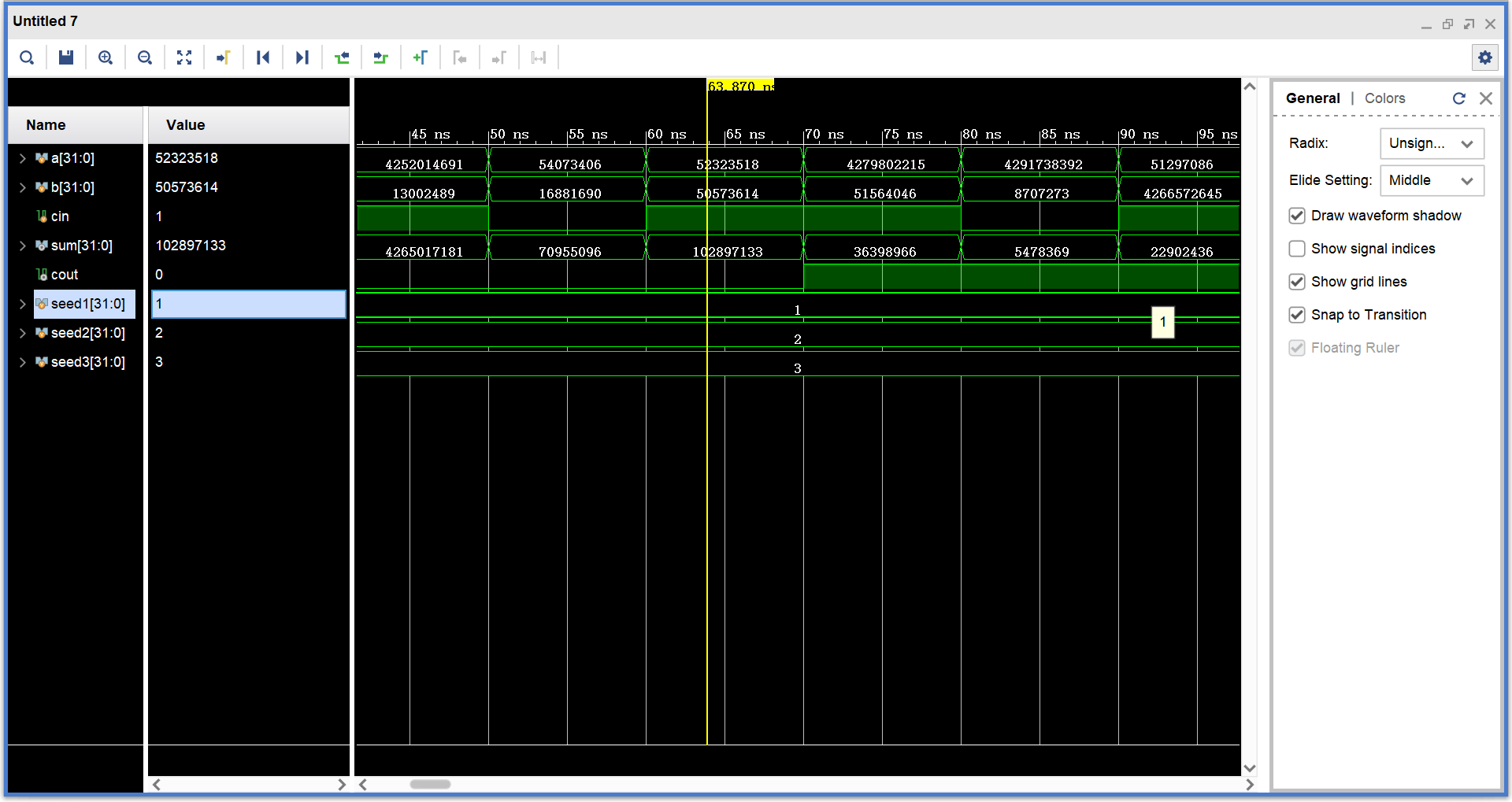展开全文verilog
• 在本文中，我们提出了一种应用驱动的ALU设计方法，以实现现代微处理器的高能效水平。我们介绍了一种PN选择算法（PNSA），使设计人员可以根据对动态电路的详细分析，为不同的应用选择高能效的动态模块。在ISCAS85和74...
• 讲述了基于资源共享的ALU设计，其中有ALU设计的基础原理及其相应图形解读。
• 华中科技大学计算机组成原理实验记录 32位ALU设计实验（运算器设计) circ文件 可直接执行。
• EDA 算术逻辑单元ALU设计 超前进位加法减法器 设计思路 VHD代码 注释仿真
• 具有乘法辅助功能的算术ALU设计 有用的可以下来看看
• 多功能ALU设计实验 一、实验目的与要求 实验目的： （1）学习多功能ALU的工作原理，掌握运算器的设计方法 （2）掌握运用Verilog HDL 进行行为描述与建模的技巧和方法 实验要求：本实验要求设计一个具有8种运算...
多功能ALU设计实验
一、实验目的与要求

实验目的：
（1）学习多功能ALU的工作原理，掌握运算器的设计方法
（2）掌握运用Verilog HDL 进行行为描述与建模的技巧和方法

实验要求：本实验要求设计一个具有8种运算功能的32位ALU，并能够产生运算结果的标志：结果为零标志ZF(Zero Flag)、溢出标志OF(Overflow Flag)。ALU通过3根控制线ALU_OP[2:0]来选择其8种功能。

功能表：

ALU_OP[2:0]
ALU_OP[2:0]
功能说明

0000
and
按位与运算

0001
or
按位或运

0010
xor
按位异或运算

0011
nor
按位或非运算

0100
add
算术加运算

0101
sub
算术减运算

0110
slt
若A<B，则输出1;否则输出0

0111
sll
B逻辑左移A所指定的位数

二、实验设计与程序代码
module ALU(OP,A,B,F,ZF,CF,OF,SF,PF);
parameter SIZE = 32;//运算位数
input [3:0] OP;//运算操作
input [SIZE:1] A;//左运算数
input [SIZE:1] B;//右运算数
output [SIZE:1] F;//运算结果
output  ZF, //0标志位, 运算结果为0(全零)则置1, 否则置0
CF, //进借位标志位, 取最高位进位C,加法时C=1则CF=1表示有进位,减法时C=0则CF=1表示有借位
OF, //溢出标志位，对有符号数运算有意义，溢出则OF=1，否则为0
SF, //符号标志位，与F的最高位相同
PF; //奇偶标志位，F有奇数个1，则PF=1，否则为0
reg [SIZE:1] F;
reg C,ZF,CF,OF,SF,PF;//C为最高位进位
always@(*)
begin
C=0;
case(OP)
4'b0000:begin F=A&B; end    //按位与
4'b0001:begin F=A|B; end    //按位或
4'b0010:begin F=A^B; end    //按位异或
4'b0011:begin F=~(A|B); end //按位或非
4'b0100:begin {C,F}=A+B; end //加法
4'b0101:begin {C,F}=A-B; end //减法
4'b0110:begin F=A<B; end    //A<B则F=1，否则F=0
4'b0111:begin F=B<<A; end   //将B左移A位
endcase
ZF = F==0;//F全为0，则ZF=1
CF = C; //进位借位标志
OF = A[SIZE]^B[SIZE]^F[SIZE]^C;//溢出标志公式
SF = F[SIZE];//符号标志,取F的最高位
PF = ~^F;//奇偶标志，F有奇数个1，则F=1；偶数个1，则F=0
end
endmodule


三、实验仿真
仿真代码👇
module Test();
reg [31:0] A,B;
reg [3:0] OP;
initial//初始化，每中运算擦拭两组数据
begin
/*按位与*/
OP=4'b0000;A=32'h0000_0000; B=32'h0000_0001;#50;
OP=4'b0000;A=32'h0000_0001; B=32'h0000_0001;#50;
/*按位或*/
OP=4'b0001;A=32'h0000_0000; B=32'h0000_0001;#50;
OP=4'b0001;A=32'h0000_0000; B=32'h0000_0000;#50;
/*按位异或*/
OP=4'b0010;A=32'h0000_0000; B=32'h0000_0001;#50;
OP=4'b0010;A=32'h0000_0000; B=32'h0000_0000;#50;
/*按位或非*/
OP=4'b0011;A=32'h0000_0000; B=32'h0000_0001;#50;
OP=4'b0011;A=32'h0000_0000; B=32'h0000_0000;#50;
/*算术加运算*/
OP=4'b0100;A=32'h7FFF_FFFF; B=32'h7FFF_FFFF;#50;
OP=4'b0100;A=32'hFFFF_FFFF; B=32'hFFFF_FFFF;#50;
/*算术减运算*/
OP=4'b0101;A=32'h7FFF_FFFF; B=32'h7FFF_FFFD;#50;
OP=4'b0101;A=32'h7FFF_FFFF; B=32'hFFFF_FFFF;#50;
/*A<B时,输出1，否则输出0*/
OP=4'b0110;A=32'h7FFF_FFFF; B=32'h8FFF_FFFF;#50;
OP=4'b0110;A=32'hFFFF_FFFF; B=32'h7FFF_FFFF;#50;
/*B逻辑左移A指定的位数*/
OP=4'b0111;A=32'h0000_0001; B=32'h0000_0001;#50;
OP=4'b0111;A=32'h0000_0001; B=32'h0000_0008;#50;
end
wire [31:0] F;
wire ZF, CF, OF, SF, PF;
ALU ALU_test(
.OP(OP),
.A(A),
.B(B),
.F(F),
.ZF(ZF),
.CF(CF),
.OF(OF),
.SF(SF),
.PF(PF)
);

endmodule


仿真波形👇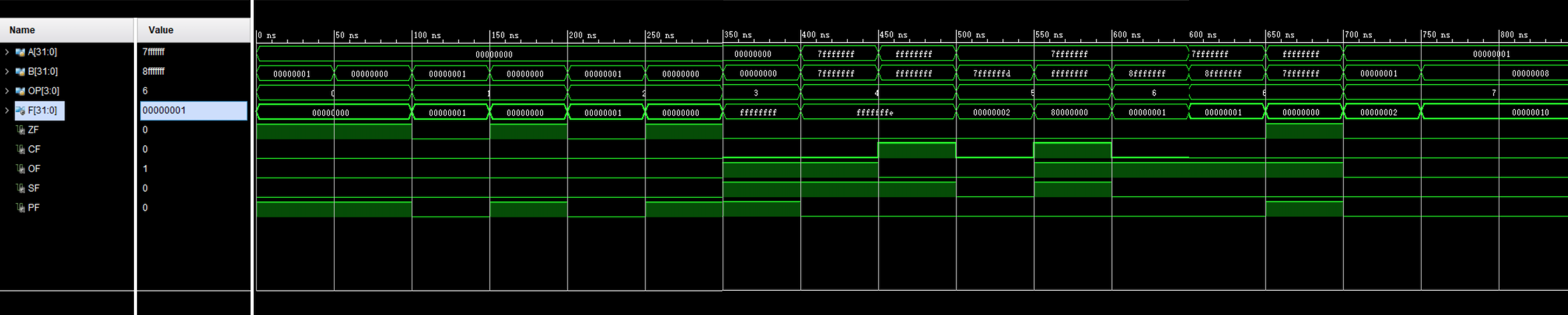四、电路图
展开全文• module ALU( input [3:0] op, input [31:0] rs1, input [31:0] rs2, input [19:0] imm, output [31:0] rd, output overflow ); wire [31:0]out1; wire [31:0]out2; wire [31:0]out3; wire [31:0]out4; wir....
• 本资源是用Verilog语言书写的32位ARM的ALU设计，FPGA实现。
• 华中科技大学计算机组成原理实验二运算器实验Logisim源文件，里面有8位可控加减法器设计、32位算术逻辑运算单元ALU设计、四位先行进位74182、四位快速加法器 、8位快速加法器、16位快速加法器、5位阵列乘法、6位补码...
• 设计思路及代码 module ALU32( ALUcont,A,B, zero,result ); input [31:0] A,B; input [2:0] ALUcont; output reg[31:0] result; output reg zero; always@(*) begin case(ALUcont) //AND 3'b000: begin ...verilog
• 用Verilog HDL设计一个模块，该模块实现了一个4bit的ALU，可以对两个4bit二进制操作数进行算术运算和逻辑运算 　算术运算包括加法与减法 　逻辑运算包括与运算、或运算 　设计一个模块，利用Verilog HDL模块元件实例...
• 这是用Verilog语言写的ARM的ALU设计，可以完成全部的16条算术指令，如加、减、反向减、带进位加等
• 用VHDL语言，模块化设计方式，实现8位运算器单元ALU设计
• 计算机组成原理上机报告，用Verilog语言实现多功能运算器ALU设计实验，仿真波形并书写实验报告。编程环境：Vivado HSL，设计语言：Verilog HDL。文档排版：LaTeX。内附实现代码，仿真波形截图，完整文档TeX源文件...
• 这是上海大学某位的论文，关于32位同时多线程微处理器的ALU设计
• ALU原理及代码 包括加法、减法、乘法、布尔算法等
• 这个压缩包里面有logisim软件和课设的电路图。ALU里面的每个器件我都画了，没有汇总，请各位自行汇总...